Following last week’s ice water blog, this week will examine a rising trend in the pursuit of a chilled drink. In most spirits, such as an expensive whisky, it is undesirable to use ice due to the fact that when the ice melts, it dilutes the drink. Instead many use a small frozen cube of soapstone known as a whisky rock. The stones for this test weigh 26.93 g (0.95 oz) and measure .875 inches on all sides. The likely problem with using the whisky rocks compared to using ice is their difference in density. Ice has a density of 0.9167 g/cm3, which is less than water’s density of 1 g /cm3, meaning that it floats. However the whisky rocks have a density of 2.98 g /cm3, meaning they will sink. As a liquid loses its heat energy, the cold water molecules (which are more dense than warmer water molecules) will sink to the bottom. With ice in the cup this works well because the warm water rises to the top and cools when it transfers its heat energy to the ice, then subsequently sinks to the bottom again. However, with the stones the cold water just sits at the bottom and therefore the drink is likely not going to be cooled as thoroughly. This week’s blog will perform a thermal analysis on the two options and examine the validity of the soapstone whisky rocks.

Heat Transfer Experiment

Purpose

The purpose of this experiment is to examine the physical differences between the use of ice and the use of soapstone whisky rocks with the intention of cooling a drink.

Scope

The scope of this experiment is to use soapstone whisky rocks and cubes of ice to find the differences in their cooling abilities of room temperature water. The glass cup will be covered to nullify any losses to evaporation or convection. Each temperature will be measured using an Omega™ HH806AU J-type thermocouple reader. The glass cups and water will start at room temperature and then will be monitored as they cool until the ice is completely melted.

Procedure

The basic procedure for this experiment will be as follows:

• Fill the two glass cups with 7 ounces of room temperature water.
• Place the desired amount of ice cubes in one of the cups with the corresponding amount of soapstone whisky rocks in the other cup.
• Keep cup covered with thermocouple in the water, ensuring it is not touching the glass cup, the ice cubes or the soapstone whisky rocks.
• Record temperature of water with ice and with soapstone whisky rocks until ice is completely melted.

Experimental Results

• With one soapstone whisky rock the water reached a low temperature of 25.5°C in the time that it took for the ice to melt.
• After 45 seconds the water with one soapstone whisky rock remained fairly constant at an average of 25.8°C, as can be seen in Figure 1 below.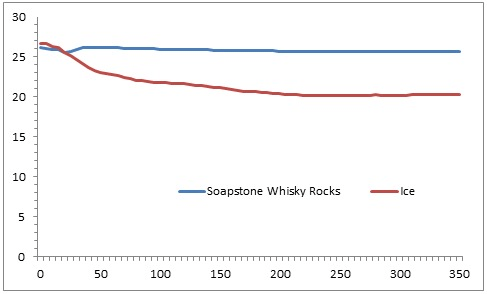Figure 1: Comparison of Water with One Soapstone Whisky Rock and One Ice Cube

• Also seen in Figure 1 is that one cube of ice cooled the water to a minimum temperature of 20.2°C.
• With two soapstone whisky rocks the water reached a low temperature of 23.6°C in the time that it took the ice to melt.
• It took 65 seconds for the water to reach a constant temperature of about 23.7°C, which is seen in Figure 2 below.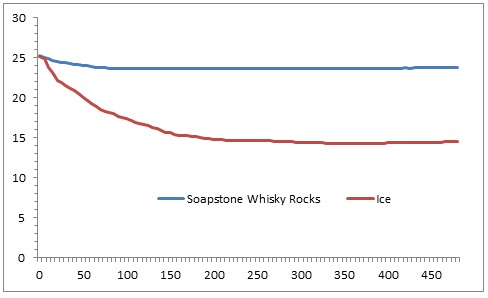Figure 2: Comparison of Water with Two Soapstone Whisky Rocks and Two Ice Cubes

• Also seen in Figure 2 is that two cubes of ice cooled the water to a minimum temperature of 14.4°C.
• With three soapstone whisky rocks the water reached a minimum temperature of 23.3°C within the time it took the ice to melt.
• It took 420 seconds for the water to remain at a constant temperature with an average of 23.7°C, as seen in Figure 3 below.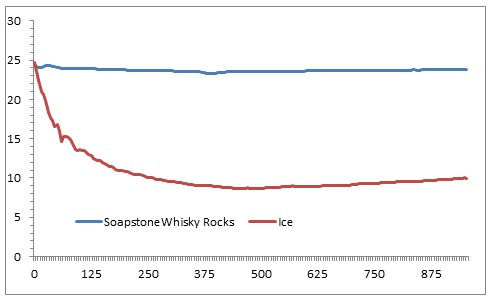Figure 3: Comparison of Water with Three Soapstone Whisky Rocks and Three Ice Cubes

• Also seen in Figure 3 is that the three cubes of ice cooled the water to a minimum temperature of 8.8°C.
• With four soapstone whisky rocks the water reached a minimum temperature of 23.2°C within the time it took for the ice to melt.
• It took 580 seconds for the water to remain at a constant temperature with an average of 23.3°C, as seen in Figure 4 below.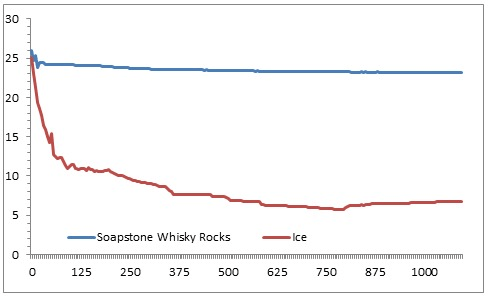Figure 4: Comparison of Water with Four  Soapstone Whisky Rocks and Four Ice Cubes

• Also seen in Figure 4 is that the four cubes of ice cooled the water to a minimum temperature of 5.8°C.

Analysis

Using the thermal mass heat transfer equation (Equation 1, seen below) the theoretical minimum temperatures were calculated for the water with soapstone whisky rocks.

T­final=(m­stone*cstone*Tinitial stone – mwater*cwater*Tinitialwater)/  (mstone*cstone+mwater*cwater)        Eqn. 1

Where m is the mass, c is the specific heat, and T is the temperature. In this scenario the mass of a soapstone whisky rock is 26.93g, the specific heat is 0.98 kJ/kg·K, the initial temperature of the soapstone whisky rocks is 9.6°C and the initial temperature of the water is 26°C. The results for the theoretical calculations can be seen in the table, Figure 5, below.

Figure 5: Theoretical Lowest Temperature of water per Soapstone Whisky Rock

[table id=whisky5 /]

The comparison of the measured lowest temperatures and the theoretical lowest temperatures can be seen in Figure 6 below.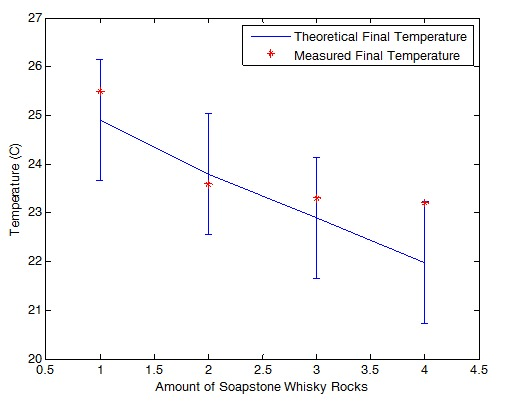Figure 6: Comparison of Theoretical and Measured Lowest Temperatures

While the measured numbers did not match up exactly with the theoretical calculations they were within the allowable error and followed the same overall trend. The error in this scenario comes from energy losses through free convection to the air and conduction to the walls of the glass cup.

When the theoretical lowest temperatures with soapstone whisky rocks are compared to the theoretical lowest temperatures of ice cubes a very large disparity is seen. The theoretical lowest temperatures of water with ice cubes are seen in Figure 7 below. These were calculated in last week’s blog using Equation 2, below.

Figure 7: Theoretical Lowest Temperature of Water per Ice Cube

[table id=whisky7 /]

mice*cice*ΔTice+nice*H+mice*cwater*ΔTmeltedice+    mwater*cwater*ΔTwater=0    Eqn. 2

Conclusions

A large difference between ice cubes and soapstone whisky rocks is a property known as thermal mass. This is essentially the amount of energy that an object would require to heat it based on its specific heat and size. When comparing ice cubes, which have a relatively low thermal mass, with soapstone whisky rocks there is a large difference in the thermal mass. This difference inhibits the soapstone whisky rocks from reaching the same initial temperature as the ice cubes, which means that it requires much less energy from the water to warm them. This is why the water did not get nearly as cold with the soapstone whisky rocks as it did with the ice cubes. If the entire mass of the soapstone whisky rocks started at 0°C as the ice cubes did then they would likely make the water much colder.

When attempting to keep your drink cold, whether it is a whisky or water, it would behoove you to use ice. If you want to avoid watering down an expensive whisky then you may have to get creative but using a soapstone whisky rock will not be as effective as ice to keep your drink cold.